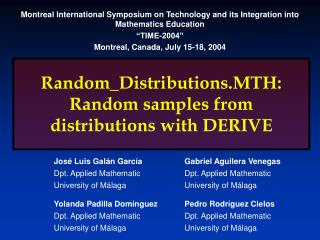Download PresentationRandom_Distributions.MTH: Random samples from distributions with DERIVERandom_Distributions.MTH: Random samples from distributions with DERIVE - PowerPoint PPT Presentation

Download PresentationRandom_Distributions.MTH: Random samples from distributions with DERIVE
An Image/Link below is provided (as is) to download presentation

Download Policy: Content on the Website is provided to you AS IS for your information and personal use and may not be sold / licensed / shared on other websites without getting consent from its author. While downloading, if for some reason you are not able to download a presentation, the publisher may have deleted the file from their server.

- - - - - - - - - - - - - - - - - - - - - - - - - - - E N D - - - - - - - - - - - - - - - - - - - - - - - - - - -
Presentation Transcript

1. Random_Distributions.MTH: Random samples from distributions with DERIVE Montreal International Symposium on Technology and its Integration into Mathematics Education “TIME-2004” Montreal, Canada, July 15-18, 2004 José Luis Galán García Dpt. Applied Mathematic University of Málaga Gabriel Aguilera Venegas Dpt. Applied Mathematic University of Málaga Yolanda Padilla Domínguez Dpt. Applied Mathematic University of Málaga Pedro Rodríguez Cielos Dpt. Applied Mathematic University of Málaga

2. INDEX • Random number generation • Derive’s random function • Ran2 algorithm • Mzran13 algorithm

3. INDEX • Random number generation • Different methods for random generation • Inverse Transform Method • Composition Method • Acceptance-rejection Method • Inverse Transform Method for discrete distributions

4. INDEX • Random number generation • Different methods for random generation • Continuous distributions • Discrete distributions • Approximative algorithms

5. Random number generationMixed congruential generators Congruential methods are based on a fundamental congruence relationship, which may be expressed as: Xi+1 = a Xi + c (mod m) Random numbers in [0,1) can be obtained by: Ui = Xi / m

6. Random number generationMixed congruential generators Full period if: • c is relative prime to m • a is congruent with 1 modulus g for every prime factor g of m • a is congruent with 1 modulus 4 if m is a multiple of 4

7. Random number generationMultiplicative and Fibonacci generators Xi+1 = a Xi (mod m) Xi+1 = a1 Xi + a2 Xi-1 + ... + ak Xi-k+1 + c (mod m) Xi+1 = a Xi Xi-1 ... Xi-k+1 + c (mod m)

8. Derive’s random generatorMixed congruential generator Xi+1 = 2.654.435.721 Xi + 1 (mod 232) It is a full period generator. Thus, its period is: 232 = 4.294.967.296

9. ran2 generatorL'Ecuyer This algorithm merges the following two multiplicative generators: Xi+1 = 40014 Xi (mod 231 - 85) Yi+1 = 40692 Yi (mod 231 - 249) Period: 2.3 1018 = 2.300.000.000.000.000.000 535.510.480 times longer than Derive’s period

10. mzran13 generatorG. Marsaglia and A. Zaman This algorithm merges the following two generators: Xi+1 = 69069 Xi + 1.013.904.243 (mod 232) Yi+1 = Yi – Yi-1 + “c” (mod 232 - 18) Period: 294 = 19.807.040.628.566.084.398.385.987.584 8.611.756.795 times longer than ran2’s period 4.611.686.018.427.387.904 than Derive’s

11. Examples

12. Random_Distributions.MTH: Random samples from distributions with DERIVE Montreal International Symposium on Technology and its Integration into Mathematics Education “TIME-2004” Montreal, Canada, July 15-18, 2004 José Luis Galán García Dpt. Applied Mathematic University of Málaga Gabriel Aguilera Venegas Dpt. Applied Mathematic University of Málaga Yolanda Padilla Domínguez Dpt. Applied Mathematic University of Málaga Pedro Rodríguez Cielos Dpt. Applied Mathematic University of Málaga